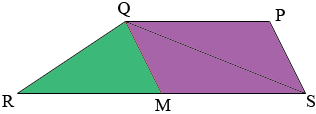SEARCH HOMEMath Central Quandaries & QueriesQuestion from rohan, a student: i have a diagram with a triangle inside a trapezoid. trapezoid is PQRS , PQ being the line above and RS THE LINE BELOW.The area of this is 12. If RS is twice PQ what is the area of PQS.Rohan,

I assume that PQ and RS are parallel. I can help you get started

1. What is the area of a trapezoid?

2. Join Q to M, the midpoint of RS.1. What do you know about triangles PQS and SQM?

2. What is the area of triangle QRM
If you need more help write back,
PennyMath Central is supported by the University of Regina and The Pacific Institute for the Mathematical Sciences.